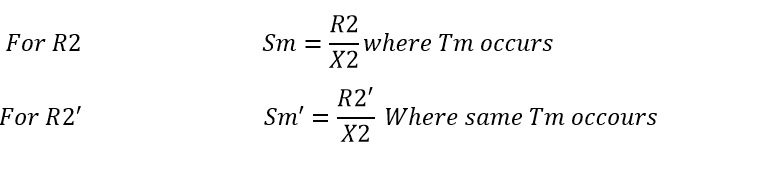# Why Slip ring Induction motor is preferred for High starting Torque application

## Why Slip ring Induction motor is preferred for High starting Torque application:

Coming to practical world, many industries operate with slip ring induction motor for high starting torque application. Example chopper, fiberizer, grinder etc. where are the high torque application is required we prepare slip ring induction motor only. In this tutorial we are going to see the functional effect of rotor resistance in slip ring induction motor.

First of all, what is slip:

Slip is nothing but the ratio of the difference between the synchronous speed to full load speed to synchronous speed. It is normally expressed in S

S = Ns-N/Ns x 100 %

Where S is Slip.

Ns= Synchronous speed.

As per torque equation:

Also see: how to drive torque equation of Slip ring induction motor

Let us consider The Torque equation at here,

Here T is motor torque

R2 is the rotor resistance

S is Slip of the motor

Rotor Voltage

Learn More:   Basic Concept of DC Generator and Types of DC generator

Also at start S=1, Then

For maximum Torque

By removing constant K

Tm Is directly proportional to square of the rotor voltage

Indirectly proportional to rotor reactance

Also condition for maximum Torque development for maximum slip

To get maximum torque at starting Rotor resistance is equal to rotor reactance.

Here R2’> R2 and the Slip Sm’ > SmDue to this, we get a new Torque-Slip characteristic of the rotor Resistance. These new characteristics is parallel to the characteristics of R2 with the same Rm but at Sm’.

The rotor resistance will be added at starting as R” like that.

For practically first they will add full resistance fo all available resistance, As preset time the resistance circuit will get cut of automatically. Finally, some resistance will be in online…

Due to this, there will be I2R loss and hence the efficiency of the motor will be poor.